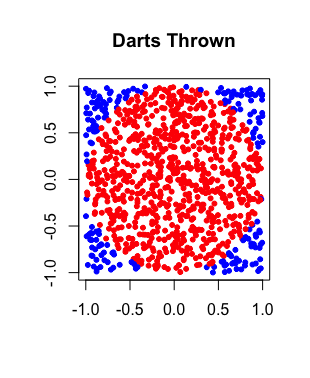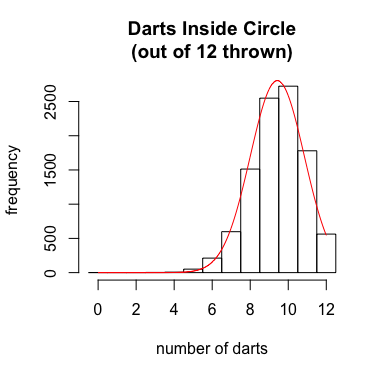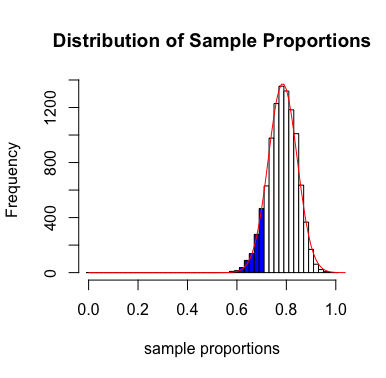R Project: DartsOne can approximate the value of $\pi$ in the following manner:

1. Throw darts randomly at a $2 \times 2$ square, centered at the origin, with bottom left corner $(-1,-1)$ and upper right corner $(1,1)$.

2. Find the proportion that land within a circle, centered at the origin, of radius 1. This proportion should, on average, be close to the ratio of the area of the circle to the area of the square -- namely, $\pi/4$.

3. Multiply this proportion by 4 to approximate the value of $\pi$.

To this end, write a function in R named num.darts.inside(n,show) that will randomly (and uniformly) choose $n$ points inside the square described above, showing these points -- if the parameter show is TRUE -- in a plot. The function should return the number of darts that landed inside the aforementioned circle of radius $1$ that is centered at the origin. Points indicating the positions of darts that landed in the circle should be colored red, while those outside the circle should be colored blue. If show is FALSE, no plot should be produced -- although the number of darts that landed in the circle should still be returned. A sample run of this function and the image it produced are shown below

> 4*num.darts.inside(1000,TRUE)/1000
 3.152Next, write a function named analysis(n) that does several things:

1. It should create a histogram that shows the distribution of the number of darts out of $n$ thrown that landed inside the circle, based on 10,000 such trials. Drawn on top of the histogram should be a red normal curve that has been vertically stretched to approximate the distribution seen in the histogram, as best as possible. There should be one bar per positive integer from $0$ to $n$, and the bars should be centered on these values. Titles for the plot and axes should be as shown below. (Note, the number shown in the main title should agree with the number of darts thrown per trial.)2. The function should calculate and print the mean number of darts seen in 10,000 trials, as well as the standard deviation, and the values that were expected for these given our assumption that the true proportion of darts expected should be $\pi/4$.

3. The function should also calculate and print the values of $np$ and $nq$, as appropriate.

4. Finally, the function should make a prediction, using the associated normal model (and appropriate continuity correction), as to what percentage of trials should result in the number of darts inside the circle being less than or equal to $n$. Of course, the actual percentage is always $100\%$, as we did not throw more than $n$ darts on any one trial. This should be indicated in your output as well.

Run your function on several values of $n$. When is the predicted percentage discussed in #4 above close to the actual percentage of $100\%$? Is there a connection between this and the values of $np$ and $nq$?

> analysis(12)
 "mean number of darts inside the circle: 9.411 (9.425 expected)"
 "standard deviation of the number of darts inside the circle: 1.43 (1.422 expected)"
 "np = 9.425"
 "nq = 2.575"
 "predicted percentage of darts using normal model <= 12: 0.9847 (expected 1.00)"

Lastly, write a function called sampling.distribution(n,p.hat) that will construct a histogram that shows the related distribution of sample proportions for darts (out of $n$ throws) that land inside the circle, again based on 10,000 trials.

This time, there should be a bar for every possible proportion that can result -- and the bars should each be centered on their corresponding proportion. Again, annotate this histogram with a red normal curve that has been vertically stretched to approximate the shape of the histogram, and title the plot and axes as shown below.

For this histogram, additionally color those bars that correspond to proportions strictly less than the value given by p.hat, and return the probability of seeing a proportion of darts inside the circle after $n$ throws less than p.hat, as approximated by an area under a normal curve. Some sample output and the corresponding image produced are shown below.

> sampling.distribution(50,0.72)
 "probability of seeing less than 0.72 is 0.13"Execute the functions analysis() and the sampling.distribution() using the same value of $n$. Do this several times, for different values of $n$. What should be concluded about when it might or might not be appropriate to calculate probabilities of seeing certain sample proportions using the normal distribution?